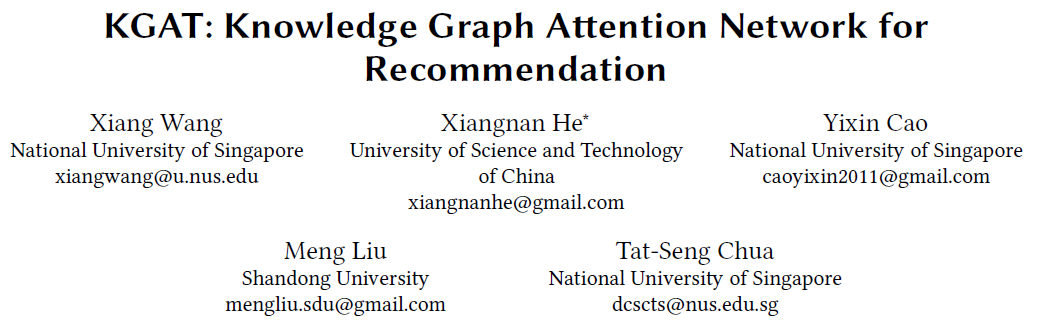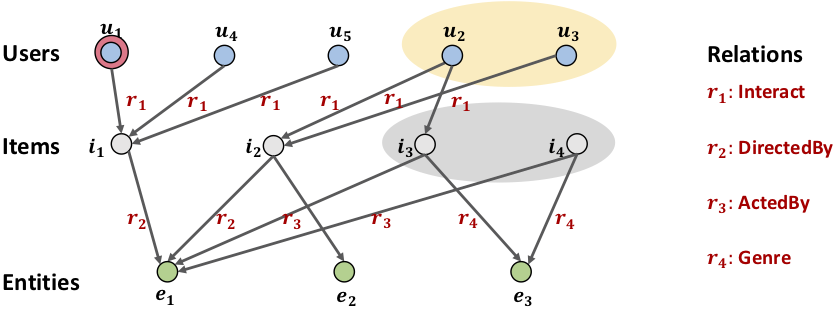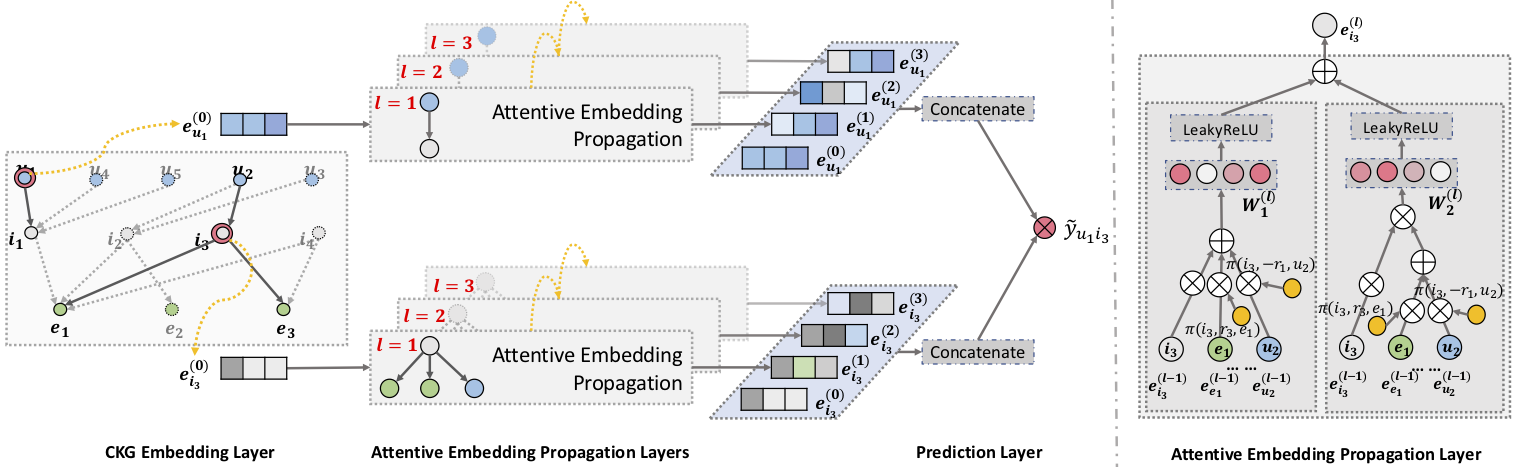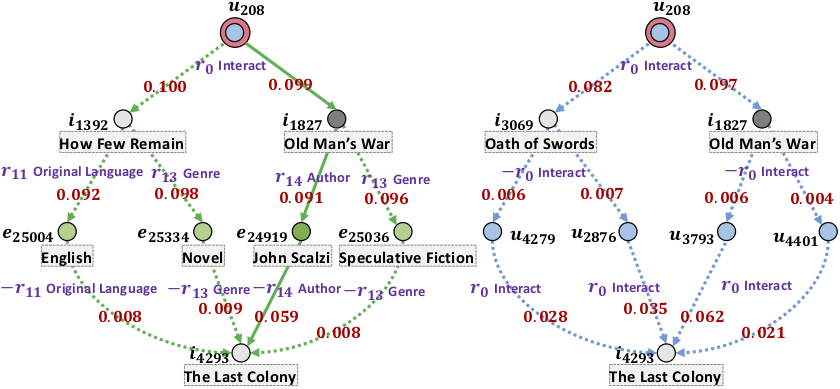# 论文阅读 KGAT: Knowledge Graph Attention Network for Recommendation## 1. Motivation## 3. Model Detailembedding：这里使用了知识图谱运用广泛的TransR方法： e h r + e r ≈ e t r \mathrm{e}_{h}^{r}+\mathrm{e}_{r} \approx \mathrm{e}_{t}^{r} 因此，可信值用如下的公式表达：
g ( h , r , t ) = ∥ W r e h + e r − W r e t ∥ 2 2 g(h, r, t)=\left\|\mathbf{W}_{r} \mathbf{e}_{h}+\mathbf{e}_{r}-\mathbf{W}_{r} \mathbf{e}_{t}\right\|_{2}^{2} 此处也是用BPR来学习关系可信度，公式就不列了。
embedding propagation: 此处的创新点在于使用attention来求每一个邻居节点的重要性。图神经网络的关键在于聚合方式，表现在两个方面，一个是邻居节点的聚合（mean，norm，plain），一个是本身和聚合邻居节点的聚合（加法，拼接，点乘）。GCN采用本身的表达加上邻居节点的聚合表达，GraphSage则采用拼接的方式，本文和NGCF类似，采用element-wise包含本身节点和邻居节点的协同过滤信号。同时，每个邻居节点应该贡献不同的权值，因此本文采用的了attention的方式来求得每一个邻居节点的重要性：
e N h = ∑ ( h , r , t ) ∈ N h π ( h , r , t ) e t \mathbf{e}_{N_{h}}=\sum_{(h, r, t) \in N_{h}} \pi(h, r, t) \mathbf{e}_{t}
π ( h , r , t ) = ( W r e t ) ⊤ tanh ⁡ ( ( W r e h + e r ) ) \pi(h, r, t)=\left(\mathrm{W}_{r} \mathrm{e}_{t}\right)^{\top} \tanh \left(\left(\mathrm{W}_{r} \mathrm{e}_{h}+\mathrm{e}_{r}\right)\right)
prediction: 另外，本文不仅仅只考虑一阶邻居，为了学到高阶信号，本文还提出了高阶传播。高阶传播都差不多，这里不赘述了。另外预测的话就是简单的用户和物品高阶表达相乘即可，最终也是使用BPR来预测。

## 4. Experiment

Attention propagation:11-01251203-011522
09-21175
04-212万+
08-282454
11-051068
07-13650
12-127920